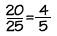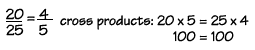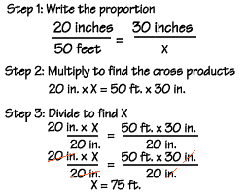Home    |    Teacher    |    Parents    |    Glossary    |    About UsA proportion is simply a statement that two ratios are equal. It can be written in two ways: as two equal fractions a/b = c/d; or using a colon, a:b = c:d. The following proportion is read as "twenty is to twenty-five as four is to five."In problems involving proportions, we can use cross products to test whether two ratios are equal and form a proportion. To find the cross products of a proportion, we multiply the outer terms, called the extremes, and the middle terms, called the means.

Here, 20 and 5 are the extremes, and 25 and 4 are the means. Since the cross products are both equal to one hundred, we know that these ratios are equal and that this is a true proportion.We can also use cross products to find a missing term in a proportion. Here's an example. In a horror movie featuring a giant beetle, the beetle appeared to be 50 feet long. However, a model was used for the beetle that was really only 20 inches long. A 30-inch tall model building was also used in the movie. How tall did the building seem in the movie?

First, write the proportion, using a letter to stand for the missing term. We find the cross products by multiplying 20 times x, and 50 times 30. Then divide to find x. Study this step closely, because this is a technique we will use often in algebra. We are trying to get our unknown number, x, on the left side of the equation, all by itself. Since x is multiplied by 20, we can use the "inverse" of multiplying, which is dividing, to get rid of the 20. We can divide both sides of the equation by the same number, without changing the meaning of the equation. When we divide both sides by 20, we find that the building will appear to be 75 feet tall.Note that we're using the inverse of multiplying by 20-that is, dividing by 20, to get x alone on one side.

 Homework Help | Pre-Algebra | Ratios and ProportionsEmail this page to a friendSearch·  Ratios·  Proportions·  Distance, rate     and time·  Similar figuresFirst Glance In Depth Examples WorkoutProportions